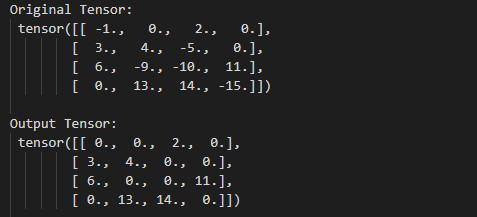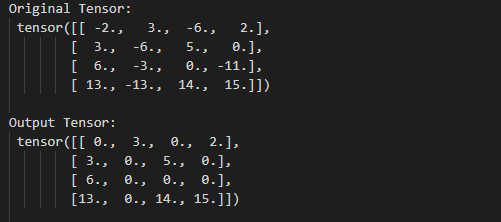# How to Apply Rectified Linear Unit Function Element-Wise in PyTorch?

• Last Updated : 02 Jun, 2022

In this article, we are going to see How to Apply Rectified Linear Unit Function Element-Wise in PyTorch in Python. We can Rectify Linear Unit Function Element-Wise by using torch.nn.ReLU() method.

## torch.nn.ReLU() method

In PyTorch, torch.nn.ReLu() method replaces all the negative values with 0 and all the non-negative left unchanged. The values of the tensor must be real only. we can also do this operation in-place by using inplace=True as a Parameter. before moving further let’s see the syntax of the given method.

Syntax: torch.nn.ReLU(inplace=False)

Parameters:

• inplace: This parameter is use when we want to do this operation in-place. Default value of inplace is False.

Example 1:

The following program is to understand how to compute the Rectified Linear Unit Function Element-Wise.

## Python

 `# Import the required library``import` `torch``import` `torch.nn as nn`` ` `# define a tensor``input` `=` `torch.tensor([[``-``1.``, ``0.``, ``2.``, ``0.``],``                      ``[``3.``, ``4.``, ``-``5.``, ``0.``],``                      ``[``6.``, ``-``9.``, ``-``10.``, ``11.``],``                      ``[``0.``, ``13.``, ``14.``, ``-``15.``]])`` ` `print``(``" Original Tensor: "``, ``input``)`` ` `# Apply Rectified Linear Unit Function ``# Element-Wise``Rel ``=` `torch.nn.ReLU()``Output ``=` `Rel(``input``)`` ` `# display result``print``(``" Output Tensor: "``, Output)`

Output:Example 2:

The following program is to understand how to Apply Rectified Linear Unit Function with inplace=True.

## Python

 `# Import the required library``import` `torch``import` `torch.nn as nn`` ` `# define a tensor``input` `=` `torch.tensor([[``-``2.``, ``3.``, ``-``6.``, ``2.``],``                      ``[``3.``, ``-``6.``, ``5.``, ``0.``],``                      ``[``6.``, ``-``3.``, ``0.``, ``-``11.``],``                      ``[``13.``, ``-``13.``, ``14.``, ``15.``]])`` ` `print``(``" Original Tensor: "``, ``input``)`` ` `# Apply Rectified Linear Unit Function ``# Element-Wise Do this operation ``# in-place``Rel ``=` `torch.nn.ReLU(inplace``=``True``)``Output ``=` `Rel(``input``)`` ` `# display result``print``(``" Output Tensor: "``, Output)`

Output:My Personal Notes arrow_drop_up